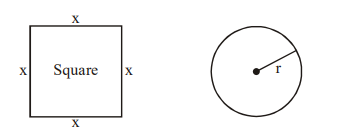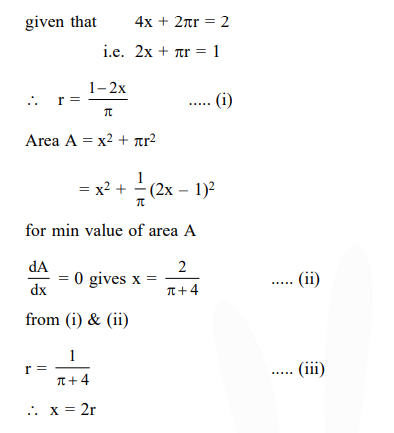# A wire of length 2 units is cut into two parts which are bent respectively`
Question:

A wire of length 2 units is cut into two parts which are bent respectively to form a square of side $=x$ units and a circle of radius $=r$ units. If the sum of the areas of the square and the circle so formed is minimum, then :

1. $2 x=r$

2. $2 \mathrm{x}=(\pi+4) \mathrm{r}$

3. $(4-\pi) \mathrm{x}=\pi \mathrm{r}$

4. $x=2 r$

Correct Option: , 4

Solution: